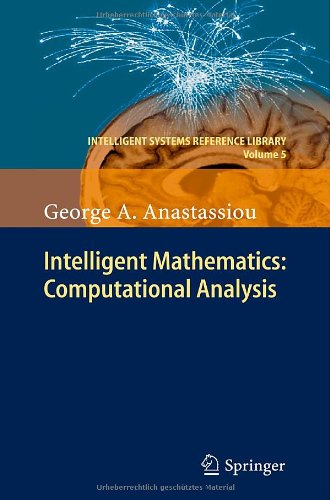# Intelligent Mathematics: Computational Analysis

• Length: 820 pages
• Edition: 2011
• Publisher:
• Publication Date: 2010-12-13
• ISBN-10: 3642170978
• ISBN-13: 9783642170973
Description

Knowledge can be modeled and computed using computational mathematical methods, then lead to real world conclusions. The strongly related to that Computational Analysis is a very large area with lots of applications. This monograph includes a great variety of topics of Computational Analysis. We present: probabilistic wavelet approximations, constrained abstract approximation theory, shape preserving weighted approximation, non positive approximations to definite integrals, discrete best approximation, approximation theory of general Picard singular operators including global smoothness preservation property, fractional singular operators. We also deal with non-isotropic general Picard singular multivariate operators and q-Gauss-Weierstrass singular q-integral operators. We talk about quantitative approximations by shift-invariant univariate and multivariate integral operators, nonlinear neural networks approximation, convergence with rates of positive linear operators, quantitative approximation by bounded linear operators, univariate and multivariate quantitative approximation by stochastic positive linear operators on univariate and multivariate stochastic processes. We further present right fractional calculus and give quantitative fractional Korovkin theory of positive linear operators. We also give analytical inequalities, fractional Opial inequalities, fractional identities and inequalities regarding fractional integrals. We further deal with semi group operator approximation, simultaneous Feller probabilistic approximation. We also present Fuzzy singular operator approximations. We give transfers from real to fuzzy approximation and talk about fuzzy wavelet and fuzzy neural networks approximations, fuzzy fractional calculus and fuzzy Ostrowski inequality. We talk about discrete fractional calculus, nabla discrete fractional calculus and inequalities. We study the q-inequalities, and q-fractional inequalities. We further study time scales: delta and nabla approaches, duality principle and inequalities. We introduce delta and nabla time scales fractional calculus and inequalities. We finally study convergence with rates of approximate solutions to exact solution of multivariate Dirichlet problem and multivariate heat equation, and discuss the uniqueness of solution of general evolution partial differential equation in multivariate time. The exposed results are expected to find applications to: applied and computational mathematics, stochastics, engineering, artificial intelligence, vision, complexity and machine learning. This monograph is suitable for graduate students and researchers.